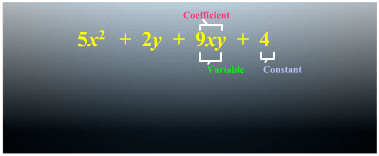# Algebraic Expressions and Identities worksheet for class 8## myCBSEguide App

CBSE, NCERT, JEE Main, NEET-UG, NDA, Exam Papers, Question Bank, NCERT Solutions, Exemplars, Revision Notes, Free Videos, MCQ Tests & more.

CBSE worksheets for Algebraic Expressions and Identities worksheet for class 8 in PDF for free download. Maths worksheets for class 8 CBSE includes worksheets on Algebraic Expressions and Identities as per NCERT syllabus. CBSE class 8 worksheets as PDF for free download Algebraic Expressions and Identities worksheets. Users can download and print the worksheets on class 8 Mathematics Algebraic Expressions and Identities for free.

# Download Algebraic Expressions and Identities worksheet for class 8

## Algebraic Expressions and Identities worksheet for class 8 Important Topics

• What are Expressions?
• Terms, Factors and Coefficients
• Monomials, Binomials and Polynomials
• Like and Unlike Terms
• Addition and Substraction of Algebraic Expressions
• Multiplication of Algebraic Expressions: Introduction
• Multiplying a Monomial by a Monomial
• Multiplying a Monomial by a Polynomial
• Multiplying a Polynomial by a Polynomial
• What is an Identity?
• Standard Identities
• Applying Identities## Some important Facts about Algebraic Expressions and Identities worksheet for class 8

1. Expressions are formed from variables and constants.
2. Terms are added to form expressions. Terms themselves are formed as product of factors.
3. Expressions that contain exactly one, two and three terms are called monomials, binomials and trinomials respectively. In general, any expression containing one or more terms with non-zero coefficients (and with variables having non- negative exponents) is called a polynomial.
4. Like terms are formed from the same variables and the powers of these variables are the same, too. Coefficients of like terms need not be the same.
5. While adding (or subtracting) polynomials, first look for like terms and add (or subtract) them; then handle the unlike terms.
6. There are number of situations in which we need to multiply algebraic expressions: for example, in finding area of a rectangle, the sides of which are given as expressions.
7. A monomial multiplied by a monomial always gives a monomial.
8. While multiplying a polynomial by a monomial, we multiply every term in the polynomial by the monomial.
9. In carrying out the multiplication of a polynomial by a binomial (or trinomial), we multiply term by term, i.e., every term of the polynomial is multiplied by every term in the binomial (or trinomial). Note that in such multiplication, we may get terms in the product which are like and have to be combined.
10.  An identity is an equality, which is true for all values of the variables in the equality. On the other hand, an equation is true only for certain values of its variables. An equation is not an identity.

## NCERT Class 8 Mathematics Solved Worksheets

• Chapter 1: Rational Numbers
• Chapter 2: Linear Equations in One Variable
• Chapter 3: Understanding Quadrilaterals
• Chapter 4: Practical Geometry
• Chapter 5: Data Handling
• Chapter 6: Squares and Square Roots
• Chapter 7: Cubes and Cube Roots
• Chapter 8: Comparing Quantities
• Chapter 9: Algebraic Expressions and Identities
• Chapter 10: Visualising Solid Shapes
• Chapter 11: Mensuration
• Chapter 12: Exponents and Powers
• Chapter 13: Direct and Inverse Proportions
• Chapter 14: Factorisation
• Chapter 15: Introduction to Graphs
• Chapter 16: Playing with Numbers

## Monomials, Binomials and Polynomials

Expression that contains only one term is called a monomial. Expression that contains two terms is called a binomial. An expressioncontaining three terms is a trinomial and so on. In general, an expression containing, one or more terms with non-zero coefficient (with variables having non negativeexponents)is called apolynomial. A polynomial may contain any number of terms, one or more than one

To download Printable worksheets for class 8 Mathematics and Science; do check myCBSEguide app or website. myCBSEguide provides sample papers with solution, test papers for chapter-wise practice, NCERT solutions, NCERT Exemplar solutions, quick revision notes for ready reference, CBSE guess papers and CBSE important question papers. Sample Paper all are made available through the best app for CBSE students and myCBSEguide website.